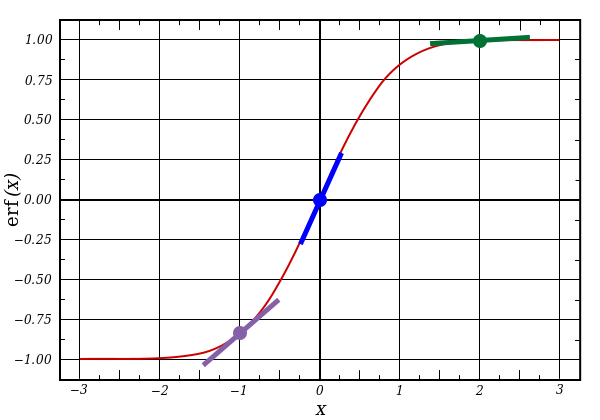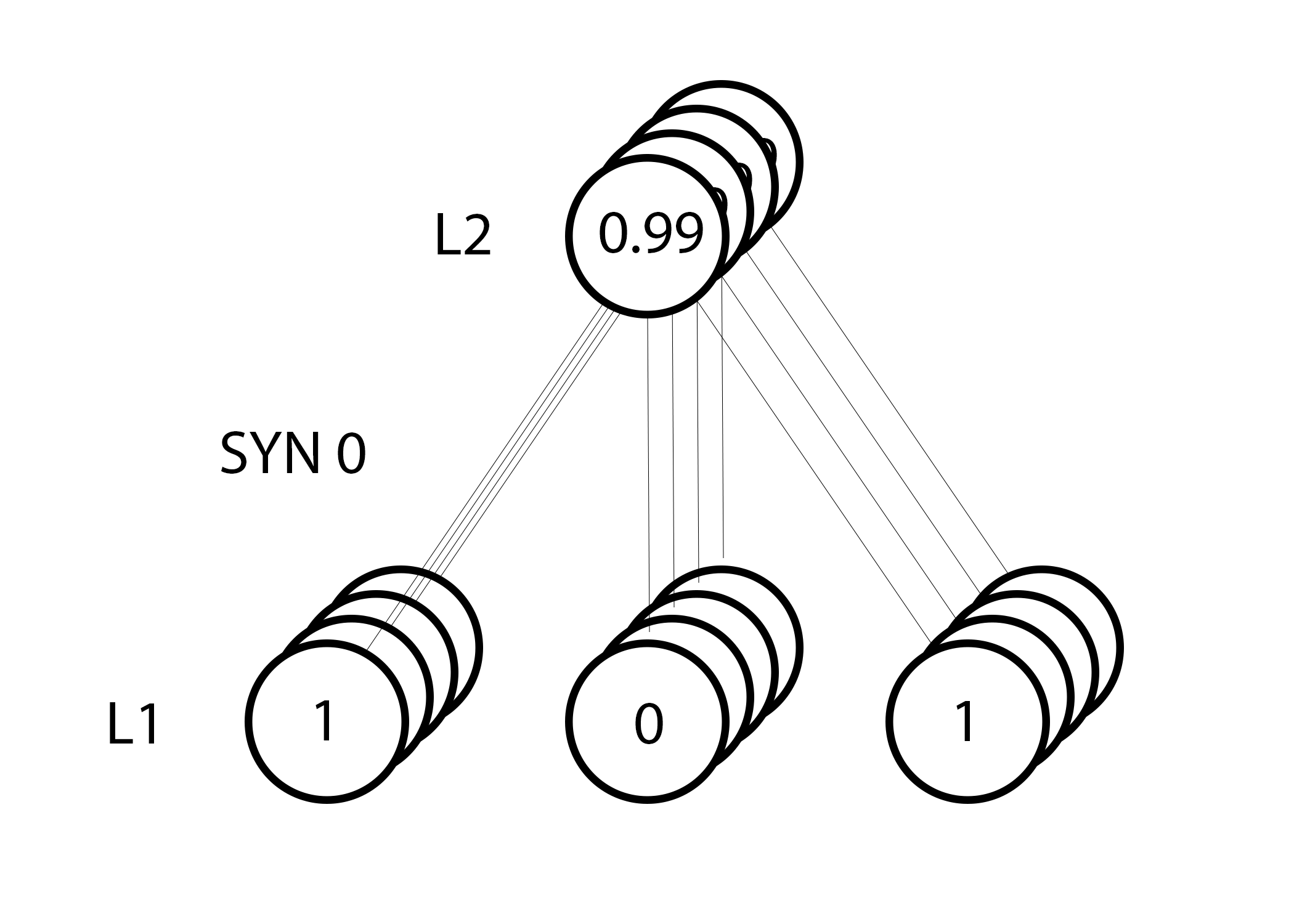# 0.写在翻译之前

本文翻译自博客：i am trask ， 属于本人一边学习神经网络一边翻译的文章。所以不止在翻译层面可能会有错误，在对神经网络的原理层面也难免会有错误。假如您发现哪里有问题，希望能谅解并留言可以让我修改，谢谢。

# 1.概要

一个能够玩的转的玩具代码对我来说是最好的学习方式。这篇教程通过一个非常简单的，Python实现的例子讲解了神经网络的反向传播算法。

X = np.array([ [0,0,1],[0,1,1],[1,0,1],[1,1,1] ])
y = np.array([[0,1,1,0]]).T
syn0 = 2*np.random.random((3,4)) - 1
syn1 = 2*np.random.random((4,1)) - 1
for j in xrange(60000):
l1 = 1/(1+np.exp(-(np.dot(X,syn0))))
l2 = 1/(1+np.exp(-(np.dot(l1,syn1))))
l2_delta = (y - l2)*(l2*(1-l2))
l1_delta = l2_delta.dot(syn1.T) * (l1 * (1-l1))
syn1 += l1.T.dot(l2_delta)
syn0 += X.T.dot(l1_delta)

# 2.神经网络的迷你玩具代码

Inputs Output
0 0 1 0
1 1 1 1
1 0 1 1
0 1 1 0

如上图，假定给了输入的三列，尝试去预测输出列的值。我们可以利用输入值与输出值进行测量统计来解决这个问题。假如这样做，我们就会发现最左边的一列完美对应输出。反向传播，以最简单的形式来说，就是像测量统计一样来生成模型。OK，下面让我们仔细研究一下。

2层神经网络：

import numpy as np

# sigmoid function
def nonlin(x,deriv=False):
if(deriv==True):
return x*(1-x)
return 1/(1+np.exp(-x))

# input dataset
X = np.array([  [0,0,1],
[0,1,1],
[1,0,1],
[1,1,1] ])

# output dataset
y = np.array([[0,0,1,1]]).T

# seed random numbers to make calculation
# deterministic (just a good practice)
np.random.seed(1)

# initialize weights randomly with mean 0
syn0 = 2*np.random.random((3,1)) - 1

for iter in xrange(10000):

# forward propagation
l0 = X
l1 = nonlin(np.dot(l0,syn0))

# how much did we miss?
l1_error = y - l1

# multiply how much we missed by the
# slope of the sigmoid at the values in l1
l1_delta = l1_error * nonlin(l1,True)

# update weights
syn0 += np.dot(l0.T,l1_delta)

print "Output After Training:"
print l1

训练后的输出：
[[ 0.00966449]
[ 0.00786506]
[ 0.99358898]
[ 0.99211957]]

X 输入数据矩阵，每行是一个训练样例
y 输出数据矩阵，每行是一个训练样例
l0 神经网络的第一层，指定为输入数据
l1 神经网络的第二层，不然的话就是隐含层
syn0 权值的第一层，突触0（Synapse0），连接l0与l1
* 元素相乘，将两个同样大小的向量对应位置的值相乘
- 元素相减，将两个同样大小的向量对应位置的值相减
x.dot(y) 如果x和y是向量，这就是一个点乘运算。如果两个都是矩阵，

正如“训练后的输出”所呈现的结果，正如我们所料！！！在我描述过程之前，我推荐你首先把玩一下上边的代码，这样你就能获得一些对于它是如何工作的玄妙的直觉。（译者注：比如可以通过在控制台输出每一步的运算结果，来理清每步的运算过程，将循环次数调整为2-5,输出程序运算过程的同时，拿出纸和笔自己笔算一遍进行验证）你可以使用ipython notebook运行上边的代码，作者吐血推荐ipython，并提供以下几点温馨提示：

• 比较第一次迭代之前与最后一次迭代之后l1的值。
• 仔细瞅瞅“nonlin”函数，这里让我们有可能得到输出。
• 在迭代的过程中，看看l1_error怎么变化的。
• 拆解36行，很多奥秘隐藏于此。
• 注意39行，神经网络中的一切都为这行操作服务。

Line 01:这一行导入了线性代数库numpy，这是本程序唯一的外部依赖。

Line 04:这是我们的“非线性函数”。这个非线性函数可以定义为不同的函数，本程序中的函数是一个叫做“sigmoid”的函数。sigmoid函数可以将任意值映射到0与1的区间内。我们使用它将数值转换为概率。sigmoid函数同样有一些其他的优良特性来满足神经网络的训练。Line 05:需要注意的是这个函数同样可以产生sigmoid函数的导数（当 deriv=Ture时）。其中一个sigmoid函数的优良特性就是，sigmoid函数在某点输出值可以用来计算其导数值。如果sigmoid的输出是一个变量“out”，那么导数值就是 “out*(1-out)”。这会使计算非常高效。

Line 10:这里初始化了我们的输入数据，作为一个numpy的矩阵。每一行就是一个“训练样例”。每列对应一个输入节点。如此以来，我们就有了3个输入节点以及4个训练样例。

Line 16:这里初始化了我们的输出集合。在这里，我生成了一个水平的集合，包含1行4列。“T”是转置函数，转置之后，y矩阵就有了4行1列。和我们的输入一样，每行是一个训练样例，每列（只有一个）是一个输出节点。因此，我们的网络就有了三个输入一个输出。

Line 20:把随机数字的种子设为一个确定值是一个好习惯。我们的数字仍然会随机分布但是每次随机生成的方式都是一样的。这样就会让我们更容易的观察网络的改变。

Line 23:这是神经网络的权值矩阵。它叫做“syn0”表示它是“0号突触（synapse zero）”。因为我们只有两层，输入与输出，所以我们只需一个权值矩阵去连接这两层。其维度是（3,1），因为我们有3个输入与一个输出。另一种方式去理解的话，l0的个数是3,l1的个数是1,同时我们需要连接所有l0与l1之间的节点，因此需要的矩阵维度是（3,1）。

Line 25:这里就开始了我们真正训练过程的代码。训练过程基于训练集迭代多次，去优化我们的神经网络。、

Line 28:因为我们的第一层，l0，就是我们的输入数据，记住X包含4个训练样例（4行）。本次的代码中我们准备要一次性的处理所有行，这种方法被称为“全批次”训练。如此以来，我们就有了4个不同的l0行，但是你可以认为这只是一个训练样例。这从本质上没有什么不同的（如果你愿意的话，再不修改代码的情况下，你也可以同时输入1000或10000行）。

Line 29:这一步用来进行预测。本质上来讲，我们首先让网络通过输入“尝试着”去预测输出。然后我们就研究怎么样每次循环都去逐步适应使得系统表现更好。

（4×3）dot(3×1)=(4×1)

Line 32:现在l1对于每个输入有了一个“猜测”的输出。现在我们可以通过用真实值y减去猜测值l1来比较“猜测”的效果。l1_error是一个包含正数与负数的向量，揭示出当前的网络到底有多少误差。

Line 36:棒棒哒！现在我们已经到了令人激动的地方啦！此行就是我们的秘密武器！这一行包含很多内容，所以让我们把它分为两部分。

（1）求导

nonlin(l1 , True)（2）完整的本行代码：误差加权导数

l1_delta = l1_error * nonlin(l1 , True)

Line 39:现在我们已经准备好更新我们的网络了！让我们拿出一个训练样例看一下。weight_update = input_value * l1_deltaInputs Output
0 0 1 0
1 1 1 1
1 0 1 1
0 1 1 0

# 4.一个难一点的问题Inputs (l0) Hidden Weights (l1) Output (l2)
0 0 1 0.1 0.2 0.5 0.2 0
0 1 1 0.2 0.6 0.7 0.1 1
1 0 1 0.3 0.2 0.3 0.9 1
1 1 1 0.2 0.1 0.3 0.8 0

import numpy as np

def nonlin(x,deriv=False):
if(deriv==True):
return x*(1-x)

return 1/(1+np.exp(-x))

X = np.array([[0,0,1],
[0,1,1],
[1,0,1],
[1,1,1]])

y = np.array([,
,
,
])

np.random.seed(1)

# randomly initialize our weights with mean 0
syn0 = 2*np.random.random((3,4)) - 1
syn1 = 2*np.random.random((4,1)) - 1

for j in xrange(60000):

# Feed forward through layers 0, 1, and 2
l0 = X
l1 = nonlin(np.dot(l0,syn0))
l2 = nonlin(np.dot(l1,syn1))

# how much did we miss the target value?
l2_error = y - l2

if (j% 10000) == 0:
print "Error:" + str(np.mean(np.abs(l2_error)))

# in what direction is the target value?
# were we really sure? if so, don't change too much.
l2_delta = l2_error*nonlin(l2,deriv=True)

# how much did each l1 value contribute to the l2 error (according to the weights)?
l1_error = l2_delta.dot(syn1.T)

# in what direction is the target l1?
# were we really sure? if so, don't change too much.
l1_delta = l1_error * nonlin(l1,deriv=True)

syn1 += l1.T.dot(l2_delta)
syn0 += l0.T.dot(l1_delta)


Error:0.496410031903
Error:0.00858452565325
Error:0.00578945986251
Error:0.00462917677677
Error:0.00395876528027
Error:0.00351012256786

Variable Definition
X 输入数据的矩阵，每行都是一个训练样例
y 输出值的矩阵，每行都是一个训练样例的输出
l0 第一层网络，节点数由输入值决定
l1 第二层网络，也叫做隐含层
l2 网络的最后一层，是我们的预测值，训练以后应该更真实值差不多
syn0 第一层权值，突触0,连接l0与l1
syn1 第二层权值，突触1,连接l1与l2
l2_error 这是神经网络输出的误差值
l2_delta 这是基于置信度衡量的输出误差，也就是说当斜率很平缓时，结果是很让人相信的
l1_error l2_delta的权值是由syn1的权值决定的，我们因此可以计算隐含层的误差
l1_delta 这是基于置信度衡量的l1的误差，同l2_delta

Line 43 : 使用l2的“可信权值误差”去建立l1的误差。其实就是把误差再通过l2到l1的连接传播过去。你可以叫这个东西为“贡献权值误差”因为我们是算了每个l1的节点值对l2的误差贡献了多少。这一步就叫做反向传播。随后我们使用跟2层实现同样的步骤去更新syn0。

# 5. 结论与进一步的工作

• Alpha
• Bias Units
• Mini-Batches
• Delta Trimming
• Parameterized Layer Sizes
• Regularization
• Dropout
• Momentum
• Batch Normalization
• GPU Compatability